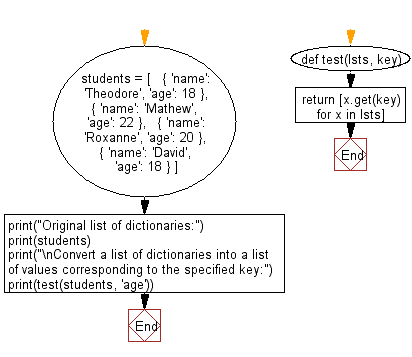﻿ Python: Convert a list of dictionaries into a list of values corresponding to the specified key - w3resource# Python: Convert a list of dictionaries into a list of values corresponding to the specified key

## Python dictionary: Exercise-73 with Solution

Write a Python program to convert a list of dictionaries into a list of values corresponding to the specified key.

• Use a list comprehension and dict.get() to get the value of key for each dictionary in lst.

Sample Solution:

Python Code:

``````def test(lsts, key):
return [x.get(key) for x in lsts]

students = [
{ 'name': 'Theodore', 'age': 18 },
{ 'name': 'Mathew', 'age': 22 },
{ 'name': 'Roxanne', 'age': 20 },
{ 'name': 'David', 'age': 18 }
]

print("Original list of dictionaries:")
print(students)
print("\nConvert a list of dictionaries into a list of values corresponding to the specified key:")
print(test(students, 'age'))
```
```

Sample Output:

```Original list of dictionaries:
[{'name': 'Theodore', 'age': 18}, {'name': 'Mathew', 'age': 22}, {'name': 'Roxanne', 'age': 20}, {'name': 'David', 'age': 18}]

Convert a list of dictionaries into a list of values corresponding to the specified key:
[18, 22, 20, 18]
```

Flowchart:## Visualize Python code execution:

The following tool visualize what the computer is doing step-by-step as it executes the said program:

Python Code Editor:

Have another way to solve this solution? Contribute your code (and comments) through Disqus.

What is the difficulty level of this exercise?

Test your Programming skills with w3resource's quiz.

﻿

## Python: Tips of the Day

Decapitalizes the first letter of a string:

Example:

```def tips_decapitalize(s, upper_rest=False):
return s[:1].lower() + (s[1:].upper() if upper_rest else s[1:])
print(tips_decapitalize('PythonTips'))
print(tips_decapitalize('PythonTips', True))
```

Output:

```pythonTips
pYTHONTIPS
```